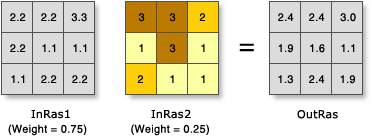# Weighted Sum (Spatial Analyst)

## Summary

Overlays several rasters, multiplying each by their given weight and summing them together.

## Illustration## Usage

• A useful way to add several rasters together is to input multiple rasters and set all weights equal to 1.

• Input rasters can be integer or floating point.

• The weight values can be any positive or negative decimal value. It is not restricted to a relative percentage nor does it need to be equal to 1.0.

• The weight will be applied to the specified field for the input raster. Fields can be of type short or long integer, double or float.

• By default, this tool will take advantage of multicore processors. The maximum number of cores that can be used is four.

To use fewer cores, use the parallelProcessingFactor environment setting.

• See Analysis environments and Spatial Analyst for additional details on the geoprocessing environments that apply to this tool.

## Syntax

`WeightedSum(in_rasters)`
 Parameter Explanation Data Type in_rasters TheWeighted Sum tool overlays several rasters, multiplying each by their given weight and summing them together.An Overlay class is used to define the table. The WSTable object is used to specify a Python list of input rasters and weight them accordingly.The form of the object is: WSTable(weightedSumTable) WSTable

#### Return Value

 Name Explanation Data Type out_raster The output weighted raster. It will be of floating-point type. Raster

## Code sample

WeightedSum example 1 (Python window)

This example creates a suitability raster for locating a ski resort by combining multiple rasters together and applying appropriate weight factors.

``````import arcpy
from arcpy import env
from arcpy.sa import *
env.workspace = "C:/sapyexamples/data"

# Execute WeightedSum
outWeightedSum = WeightedSum(WSTable([["snow", "VALUE", 0.25], ["land", "VALUE",0.25],
["soil", "VALUE", 0.5]]))
outWeightedSum.save("C:/sapyexamples/output/outwsum")``````
WeightedSum example 2 (stand-alone script)

This example creates a suitability raster for locating a ski resort by combining multiple rasters together and applying appropriate weight factors.

``````# Name: WeightedSum_Ex_02.py
# Description: Overlays several rasters multiplying each by their given
#    weight and summing them together.
# Requirements: Spatial Analyst Extension

# Import system modules
import arcpy
from arcpy import env
from arcpy.sa import *

# Set environment settings
env.workspace = "C:/sapyexamples/data"

# Set local variables
inRaster1 = "snow"
inRaster2 = "land"
inRaster3 = "soil"
WSumTableObj = WSTable([[inRaster1, "VALUE", 0.25], [inRaster2, "VALUE", 0.25],
[inRaster3, "VALUE", 0.5]])

# Execute WeightedSum
outWeightedSum = WeightedSum(WSumTableObj)

# Save the output
outWeightedSum.save("C:/sapyexamples/output/weightsumout")``````

## Licensing information

• Basic: Requires Spatial Analyst or Image Analyst
• Standard: Requires Spatial Analyst or Image Analyst
• Advanced: Requires Spatial Analyst or Image Analyst